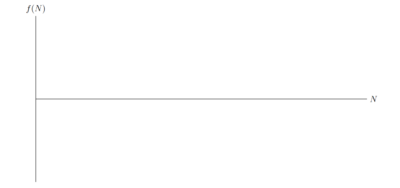# Science:Math Exam Resources/Courses/MATH102/December 2019/Question 08(a)

MATH102 December 2019

Work in progress: this question page is incomplete, there might be mistakes in the material you are seeing here.
Other MATH102 Exams

### Question 08(a)

The Allee effect is a phenomenon in ecology by which the per capita reproduction rate of the population increases with increasing population density. In Canada the Allee effect can be observed in the Atlantic cod population in the Gulf of St.Lawrence, where cod is the preferred prey of grey seals. A simple model of the Atlantic cod population growth rate is a modified logistic equation:

$\displaystyle f(N)=N({\frac {N}{2}}-1)(1-N)$where N is measured in millions of kg of fish. We can assume $N\geq 0.$(4 points) Sketch the function f(N) for $N\geq 0$. Include the N-values of any roots, extrema, inflection points, and horizontal asymptotes of the function f(N), should they exits. Make sure your graph is big enough to clearly show all intersecting points.Make sure you understand the problem fully: What is the question asking you to do? Are there specific conditions or constraints that you should take note of? How will you know if your answer is correct from your work only? Can you rephrase the question in your own words in a way that makes sense to you? If you are stuck, check the hint below. Consider it for a while. Does it give you a new idea on how to approach the problem? If so, try it!

 Checking a solution serves two purposes: helping you if, after having used the hint, you still are stuck on the problem; or if you have solved the problem and would like to check your work. If you are stuck on a problem: Read the solution slowly and as soon as you feel you could finish the problem on your own, hide it and work on the problem. Come back later to the solution if you are stuck or if you want to check your work. If you want to check your work: Don't only focus on the answer, problems are mostly marked for the work you do, make sure you understand all the steps that were required to complete the problem and see if you made mistakes or forgot some aspects. Your goal is to check that your mental process was correct, not only the result.Math Learning Centre A space to study math together. Free math graduate and undergraduate TA support. Mon - Fri: 11 am - 5 pm, Private tutor We can help to